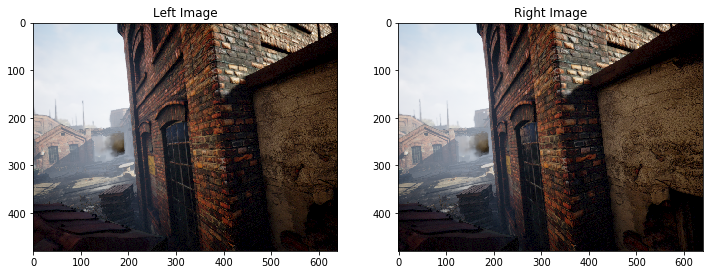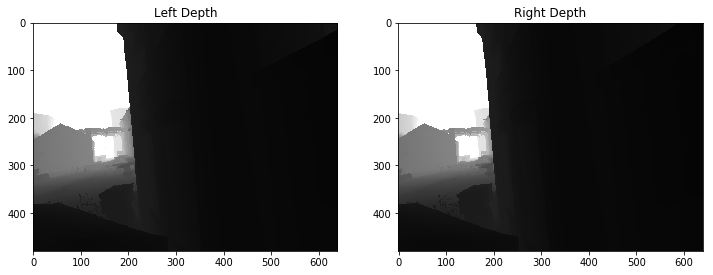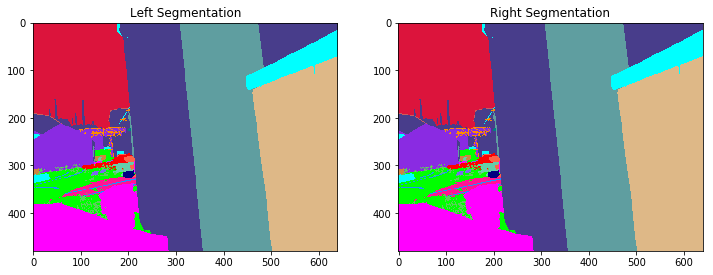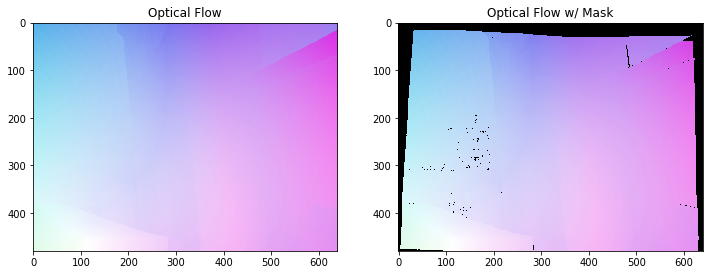# TartanAir: AirSim Simulation Dataset for Simultaneous Localization and Mapping

VSLAM AirSim Images Tartan Air• 立體影像：影像類型 (png)。

• 深度檔案：numpy 類型 (npy)。

• 分割檔案：numpy 類型 (npy)。

• 光流檔案：numpy 類型 (npy)。

• 相機姿勢偵測檔案：文字類型 (txt)。#### 高難度視覺效果

• 光線條件不佳。 晝夜替換。 光線太暗。 照明情況快速變更。
• 天氣效果。 晴天、雨天、下雪、颳風和多霧。
• 季節更迭。#### 引文

```@article{tartanair2020arxiv, title = {TartanAir: A Dataset to Push the Limits of Visual SLAM}, author = {Wenshan Wang, Delong Zhu, Xiangwei Wang, Yaoyu Hu, Yuheng Qiu, Chen Wang, Yafei Hu, Ashish Kapoor, Sebastian Scherer}, journal = {arXiv preprint arXiv:2003.14338}, year = {2020}, url = {https://arxiv.org/abs/2003.14338} } @inproceedings{airsim2017fsr, author = {Shital Shah and Debadeepta Dey and Chris Lovett and Ashish Kapoor}, title = {AirSim: High-Fidelity Visual and Physical Simulation for Autonomous Vehicles}, year = {2017}, booktitle = {Field and Service Robotics}, eprint = {arXiv:1705.05065}, url = {https://arxiv.org/abs/1705.05065} }```

#### 通知

Microsoft 係依「現況」提供 Azure 開放資料集。 針對　貴用戶對資料集的使用，Microsoft 不提供任何明示或默示的擔保、保證或條件。 在　貴用戶當地法律允許的範圍內，針對因使用資料集而導致的任何直接性、衍生性、特殊性、間接性、附隨性或懲罰性損害或損失，Microsoft 概不承擔任何責任。

### Access

Available inWhen to use
Azure Notebooks

Quickly explore the dataset with Jupyter notebooks hosted on Azure or your local machine.

Select your preferred service:

Azure Notebooks

### Azure Notebooks

Package: Language: Python

## Demo notebook for accessing TartanAir data on Azure¶

!! NOTE: This sample file should only be used on Azure. To download the data to your local machine, please refer to the website: http://theairlab.org/tartanair-dataset/

This notebook provides an example of accessing TartanAir data from blobl storage on Azure, including:

1) navigate the directories of different environments and trajectories.

2) load the data into memory, and

3) visualize the data.

## Data directory structure¶

``````ROOT
|
--- ENV_NAME_0                             # environment folder
|       |
|       ---- Easy                          # difficulty level
|       |      |
|       |      ---- P000                   # trajectory folder
|       |      |      |
|       |      |      +--- depth_left      # 000000_left_depth.npy - 000xxx_left_depth.npy
|       |      |      +--- depth_right     # 000000_right_depth.npy - 000xxx_right_depth.npy
|       |      |      +--- flow            # 000000_000001_flow/mask.npy - 000xxx_000xxx_flow/mask.npy
|       |      |      +--- image_left      # 000000_left.png - 000xxx_left.png
|       |      |      +--- image_right     # 000000_right.png - 000xxx_right.png
|       |      |      +--- seg_left        # 000000_left_seg.npy - 000xxx_left_seg.npy
|       |      |      +--- seg_right       # 000000_right_seg.npy - 000xxx_right_seg.npy
|       |      |      ---- pose_left.txt
|       |      |      ---- pose_right.txt
|       |      |
|       |      +--- P001
|       |      .
|       |      .
|       |      |
|       |      +--- P00K
|       |
|       +--- Hard
|
+-- ENV_NAME_1
.
.
|
+-- ENV_NAME_N``````

## Notebook dependencies¶

`pip install numpy`

`pip install azure-storage-blob`

`pip install opencv-python`

## Imports and contrainer_client¶

In :
```from azure.storage.blob import ContainerClient
import numpy as np
import io
import cv2
import time
import matplotlib.pyplot as plt
%matplotlib inline

# Dataset website: http://theairlab.org/tartanair-dataset/
account_url = 'https://tartanair.blob.core.windows.net/'
container_name = 'tartanair-release1'

container_client = ContainerClient(account_url=account_url,
container_name=container_name,
credential=None)
```

## List the environments and trajectories¶

In :
```def get_environment_list():
'''
List all the environments shown in the root directory
'''
env_gen = container_client.walk_blobs()
envlist = []
for env in env_gen:
envlist.append(env.name)
return envlist

def get_trajectory_list(envname, easy_hard = 'Easy'):
'''
List all the trajectory folders, which is named as 'P0XX'
'''
assert(easy_hard=='Easy' or easy_hard=='Hard')
traj_gen = container_client.walk_blobs(name_starts_with=envname + '/' + easy_hard+'/')
trajlist = []
for traj in traj_gen:
trajname = traj.name
trajname_split = trajname.split('/')
trajname_split = [tt for tt in trajname_split if len(tt)>0]
if trajname_split[-1] == 'P':
trajlist.append(trajname)
return trajlist

def _list_blobs_in_folder(folder_name):
"""
List all blobs in a virtual folder in an Azure blob container
"""

files = []
generator = container_client.list_blobs(name_starts_with=folder_name)
for blob in generator:
files.append(blob.name)
return files

def get_image_list(trajdir, left_right = 'left'):
assert(left_right == 'left' or left_right == 'right')
files = _list_blobs_in_folder(trajdir + '/image_' + left_right + '/')
files = [fn for fn in files if fn.endswith('.png')]
return files

def get_depth_list(trajdir, left_right = 'left'):
assert(left_right == 'left' or left_right == 'right')
files = _list_blobs_in_folder(trajdir + '/depth_' + left_right + '/')
files = [fn for fn in files if fn.endswith('.npy')]
return files

def get_flow_list(trajdir, ):
files = _list_blobs_in_folder(trajdir + '/flow/')
files = [fn for fn in files if fn.endswith('flow.npy')]
return files

def get_flow_mask_list(trajdir, ):
files = _list_blobs_in_folder(trajdir + '/flow/')
files = [fn for fn in files if fn.endswith('mask.npy')]
return files

def get_posefile(trajdir, left_right = 'left'):
assert(left_right == 'left' or left_right == 'right')
return trajdir + '/pose_' + left_right + '.txt'

def get_seg_list(trajdir, left_right = 'left'):
assert(left_right == 'left' or left_right == 'right')
files = _list_blobs_in_folder(trajdir + '/seg_' + left_right + '/')
files = [fn for fn in files if fn.endswith('.npy')]
return files
```

### List all the environments¶

In :
```envlist = get_environment_list()
print('Find {} environments..'.format(len(envlist)))
print(envlist)
```
```Find 18 environments..
['abandonedfactory/', 'abandonedfactory_night/', 'amusement/', 'carwelding/', 'endofworld/', 'gascola/', 'hospital/', 'japanesealley/', 'neighborhood/', 'ocean/', 'office/', 'office2/', 'oldtown/', 'seasidetown/', 'seasonsforest/', 'seasonsforest_winter/', 'soulcity/', 'westerndesert/']
```

### List all the 'Easy' trajectories in the first environment¶

In :
```diff_level = 'Easy'
env_ind = 0
trajlist = get_trajectory_list(envlist[env_ind], easy_hard = diff_level)
print('Find {} trajectories in {}'.format(len(trajlist), envlist[env_ind]+diff_level))
print(trajlist)
```
```Find 10 trajectories in abandonedfactory/Easy
['abandonedfactory/Easy/P000/', 'abandonedfactory/Easy/P001/', 'abandonedfactory/Easy/P002/', 'abandonedfactory/Easy/P004/', 'abandonedfactory/Easy/P005/', 'abandonedfactory/Easy/P006/', 'abandonedfactory/Easy/P008/', 'abandonedfactory/Easy/P009/', 'abandonedfactory/Easy/P010/', 'abandonedfactory/Easy/P011/']
```

### List all the data files in one trajectory¶

In :
```traj_ind = 1
traj_dir = trajlist[traj_ind]

left_img_list = get_image_list(traj_dir, left_right = 'left')
print('Find {} left images in {}'.format(len(left_img_list), traj_dir))

right_img_list = get_image_list(traj_dir, left_right = 'right')
print('Find {} right images in {}'.format(len(right_img_list), traj_dir))

left_depth_list = get_depth_list(traj_dir, left_right = 'left')
print('Find {} left depth files in {}'.format(len(left_depth_list), traj_dir))

right_depth_list = get_depth_list(traj_dir, left_right = 'right')
print('Find {} right depth files in {}'.format(len(right_depth_list), traj_dir))

left_seg_list = get_seg_list(traj_dir, left_right = 'left')
print('Find {} left segmentation files in {}'.format(len(left_seg_list), traj_dir))

right_seg_list = get_seg_list(traj_dir, left_right = 'left')
print('Find {} right segmentation files in {}'.format(len(right_seg_list), traj_dir))

flow_list = get_flow_list(traj_dir)
print('Find {} flow files in {}'.format(len(flow_list), traj_dir))

flow_mask_list = get_flow_mask_list(traj_dir)
print('Find {} flow mask files in {}'.format(len(flow_mask_list), traj_dir))

left_pose_file = get_posefile(traj_dir, left_right = 'left')
print('Left pose file: {}'.format(left_pose_file))

right_pose_file = get_posefile(traj_dir, left_right = 'right')
print('Right pose file: {}'.format(right_pose_file))
```
```Find 434 left images in abandonedfactory/Easy/P001/
Find 434 right images in abandonedfactory/Easy/P001/
Find 434 left depth files in abandonedfactory/Easy/P001/
Find 434 right depth files in abandonedfactory/Easy/P001/
Find 434 left segmentation files in abandonedfactory/Easy/P001/
Find 434 right segmentation files in abandonedfactory/Easy/P001/
Find 433 flow files in abandonedfactory/Easy/P001/
Find 433 flow mask files in abandonedfactory/Easy/P001/
Left pose file: abandonedfactory/Easy/P001//pose_left.txt
Right pose file: abandonedfactory/Easy/P001//pose_right.txt
```

## Functions for data downloading¶

In :
```def read_numpy_file(numpy_file,):
'''
return a numpy array given the file path
'''
bc = container_client.get_blob_client(blob=numpy_file)
data = bc.download_blob()
ee = io.BytesIO(data.content_as_bytes())
ff = np.load(ee)
return ff

def read_image_file(image_file,):
'''
return a uint8 numpy array given the file path
'''
bc = container_client.get_blob_client(blob=image_file)
data = bc.download_blob()
ee = io.BytesIO(data.content_as_bytes())
img=cv2.imdecode(np.asarray(bytearray(ee.read()),dtype=np.uint8), cv2.IMREAD_COLOR)
im_rgb = img[:, :, [2, 1, 0]] # BGR2RGB
return im_rgb
```

## Functions for data visualization¶

In :
```def depth2vis(depth, maxthresh = 50):
depthvis = np.clip(depth,0,maxthresh)
depthvis = depthvis/maxthresh*255
depthvis = depthvis.astype(np.uint8)
depthvis = np.tile(depthvis.reshape(depthvis.shape+(1,)), (1,1,3))

return depthvis

def seg2vis(segnp):
colors = [(205, 92, 92), (0, 255, 0), (199, 21, 133), (32, 178, 170), (233, 150, 122), (0, 0, 255), (128, 0, 0), (255, 0, 0), (255, 0, 255), (176, 196, 222), (139, 0, 139), (102, 205, 170), (128, 0, 128), (0, 255, 255), (0, 255, 255), (127, 255, 212), (222, 184, 135), (128, 128, 0), (255, 99, 71), (0, 128, 0), (218, 165, 32), (100, 149, 237), (30, 144, 255), (255, 0, 255), (112, 128, 144), (72, 61, 139), (165, 42, 42), (0, 128, 128), (255, 255, 0), (255, 182, 193), (107, 142, 35), (0, 0, 128), (135, 206, 235), (128, 0, 0), (0, 0, 255), (160, 82, 45), (0, 128, 128), (128, 128, 0), (25, 25, 112), (255, 215, 0), (154, 205, 50), (205, 133, 63), (255, 140, 0), (220, 20, 60), (255, 20, 147), (95, 158, 160), (138, 43, 226), (127, 255, 0), (123, 104, 238), (255, 160, 122), (92, 205, 92),]
segvis = np.zeros(segnp.shape+(3,), dtype=np.uint8)

for k in range(256):
mask = segnp==k
colorind = k % len(colors)
if np.sum(mask)>0:
segvis[mask,:] = colors[colorind]

return segvis

def _calculate_angle_distance_from_du_dv(du, dv, flagDegree=False):
a = np.arctan2( dv, du )

angleShift = np.pi

if ( True == flagDegree ):
a = a / np.pi * 180
angleShift = 180
# print("Convert angle from radian to degree as demanded by the input file.")

d = np.sqrt( du * du + dv * dv )

return a, d, angleShift

def flow2vis(flownp, maxF=500.0, n=8, mask=None, hueMax=179, angShift=0.0):
"""
Show a optical flow field as the KITTI dataset does.
Some parts of this function is the transform of the original MATLAB code flow_to_color.m.
"""

ang, mag, _ = _calculate_angle_distance_from_du_dv( flownp[:, :, 0], flownp[:, :, 1], flagDegree=False )

# Use Hue, Saturation, Value colour model
hsv = np.zeros( ( ang.shape, ang.shape, 3 ) , dtype=np.float32)

am = ang < 0
ang[am] = ang[am] + np.pi * 2

hsv[ :, :, 0 ] = np.remainder( ( ang + angShift ) / (2*np.pi), 1 )
hsv[ :, :, 1 ] = mag / maxF * n
hsv[ :, :, 2 ] = (n - hsv[:, :, 1])/n

hsv[:, :, 0] = np.clip( hsv[:, :, 0], 0, 1 ) * hueMax
hsv[:, :, 1:3] = np.clip( hsv[:, :, 1:3], 0, 1 ) * 255
hsv = hsv.astype(np.uint8)

rgb = cv2.cvtColor(hsv, cv2.COLOR_HSV2RGB)

if ( mask is not None ):
mask = mask > 0
rgb[mask] = np.array([0, 0 ,0], dtype=np.uint8)

return rgb
```

### Download and visualize the data¶

In :
```data_ind = 173 # randomly select one frame (data_ind < TRAJ_LEN)
```

#### Visualize the left and right RGB images¶

In :
```left_img = read_image_file(left_img_list[data_ind])
right_img = read_image_file(right_img_list[data_ind])

plt.figure(figsize=(12, 5))
plt.subplot(121)
plt.imshow(left_img)
plt.title('Left Image')
plt.subplot(122)
plt.imshow(right_img)
plt.title('Right Image')
plt.show()
```#### Visualize the left and right depth files¶

In :
```left_depth = read_numpy_file(left_depth_list[data_ind])
left_depth_vis = depth2vis(left_depth)

right_depth = read_numpy_file(right_depth_list[data_ind])
right_depth_vis = depth2vis(right_depth)

plt.figure(figsize=(12, 5))
plt.subplot(121)
plt.imshow(left_depth_vis)
plt.title('Left Depth')
plt.subplot(122)
plt.imshow(right_depth_vis)
plt.title('Right Depth')
plt.show()
```#### Visualize the left and right segmentation files¶

In :
```left_seg = read_numpy_file(left_seg_list[data_ind])
left_seg_vis = seg2vis(left_seg)

right_seg = read_numpy_file(right_seg_list[data_ind])
right_seg_vis = seg2vis(right_seg)

plt.figure(figsize=(12, 5))
plt.subplot(121)
plt.imshow(left_seg_vis)
plt.title('Left Segmentation')
plt.subplot(122)
plt.imshow(right_seg_vis)
plt.title('Right Segmentation')
plt.show()
```#### Visualize the flow and mask files¶

In :
```flow = read_numpy_file(flow_list[data_ind])
flow_vis = flow2vis(flow)

flow_mask = read_numpy_file(flow_mask_list[data_ind])
flow_vis_w_mask = flow2vis(flow, mask = flow_mask)

plt.figure(figsize=(12, 5))
plt.subplot(121)
plt.imshow(flow_vis)
plt.title('Optical Flow')
plt.subplot(122)
plt.imshow(flow_vis_w_mask)
plt.title('Optical Flow w/ Mask')
plt.show()
```